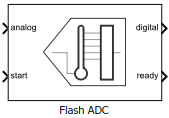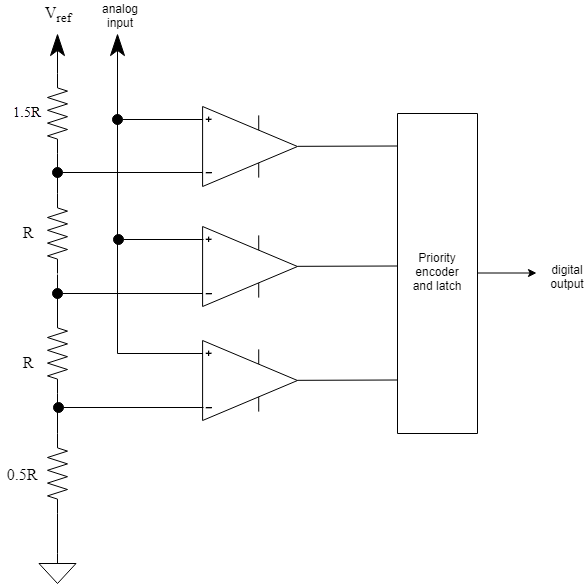Documentation

• Library:
• Mixed-Signal Blockset / ADC / Architectures## Description

An N-bit flash ADC comprises of a resistive ladder that contains 2N resistors and 2N-1 comparators.The reference voltage of each comparator is 1 least significant bit (LSB) higher than the one below it in the ladder. As a result, all comparators below a certain point will have input voltage greater than the reference voltage, and a logic `1` output. All comparators above that point will have input voltage smaller than the reference voltage, and a logic `0` output. The output of 2N-1 comparators are passed through a priority encoder to produce the digital output. This encoding scheme is called thermometer encoding.

Since the analog input is applied to all the comparators at once, the flash ADC architecture is very fast. But the ADC has low resolution and high power requirements due to a large number of resistors required to implement the architecture.

## Ports

### Input

expand all

Data Types: `double`

External conversion start clock, specified as a scalar or vector. start determines when the analog to digital conversion process starts.

Data Types: `double`

### Output

expand all

Converted digital output signal, returned as scalar or vector.

Data Types: `fixed point` | `single` | `double` | `uint8` | `uint16` | `uint32` | `Boolean`

Determines whether ADC conversion is complete, returned as a scalar or vector.

Data Types: `single` | `double` | `uint8` | `uint16` | `uint32` | `fixed point`

## Parameters

expand all

### Configuration

Number of physical output bits, specified as a unitless positive real integer. Number of bits determines the resolution of the ADC.

#### Programmatic Use

• Use `get_param(gcb,'NBits')` to view the current Number of bits.

• Use `set_param(gcb,'NBits',value)` to set Number of bits to a specific value.

Data Types: `double`

ADC dynamic range, specified as a 2-element row vector in volts.

#### Programmatic Use

• Use `get_param(gcb,'InputRange')` to view the current Input range (V).

• Use `set_param(gcb,'InputRange',value)` to set Input range (V) to a specific value.

Data Types: `double`

Select to connect to an external start conversion clock. By default, this option is selected. If you deselect this option, a Sampling Clock Source block inside the Flash ADC is used to generate the start conversion clock.

Frequency of internal start conversion clock, specified as a positive real scalar in Hz. Conversion start frequency determines the rate of the ADC.

#### Dependencies

This parameter is only available when Use external start clock is not selected.

#### Programmatic Use

• Use `get_param(gcb,'StartFreq')` to view the current value of Conversion start frequency (Hz).

• Use `set_param(gcb,'StartFreq',value)` to set Conversion start frequency (Hz) to a specific value.

Data Types: `double`

RMS aperture jitter added as an impairment to the start conversion clock, specified as a real nonnegative scalar in s. Set RMS aperture jitter value to zero if you want a clean clock signal.

#### Dependencies

This parameter is only available when Use external start clock is not selected.

#### Programmatic Use

• Use `get_param(gcb,'StartClkJitter')` to view the current value of RMS aperture jitter (s).

• Use `set_param(gcb,'StartClkJitter',value)` to set RMS aperture jitter (s) to a specific value.

Data Types: `double`

Clock edge type that triggers the output update:

• `Rising edge` — the output is updated with the rising edge of the clock signal.

• `Falling edge` — the output is updated with the falling edge of the clock signal.

• `Either edge` — the output is updated with both the rising and the falling edge of the clock signal.

#### Programmatic Use

• Use `get_param(gcb,'Trigger')` to view the current Edge trigger type.

• Use `set_param(gcb,'Trigger',value)` to set Edge trigger type to a specific value.

#### Programmatic Use

• Use `get_param(gcb,'OutDataType')` to view the current ADC output data type.

• Use `set_param(gcb,'OutDataType',value)` to set ADC output data type to a specific value.

### Impairments

Select to enable impairments such as offset error and gain error in ADC simulation. By default, this option is selected.

Shifts quantization steps by specific value, specified as a scalar in least significant bit (LSB).

#### Dependencies

This parameter is only available when Enable impairments is selected.

#### Programmatic Use

• Use `get_param(gcb,'OffsetError')` to view the current value of Offset error (LSB).

• Use `set_param(gcb,'OffsetError',value)` to set Offset error (LSB) to a specific value.

Data Types: `double`

Error on the slope of the straight line interpolating ADC transfer curve, specified as a real scalar in LSB or %.

#### Dependencies

This parameter is only available when Enable impairments is selected.

#### Programmatic Use

• Use `get_param(gcb,'GainError')` to view the current value of Gain error.

• Use `set_param(gcb,'GainError',value)` to set Gain error to a specific value.

Data Types: `double`

Position of the failed comparators, specified as a row vector with positive real values.

#### Dependencies

This parameter is only available when Enable impairments is selected.

#### Programmatic Use

• Use `get_param(gcb,'Bubbles')` to view the current Missing codes.

• Use `set_param(gcb,'Bubbles',value)` to set Missing codes to a specific value.

Data Types: `double`